# Graphical Division Geometry For CAT

Most area based geometry questions can be solved without using formulas and without consuming precious time by graphically dividing the figure. Let us understand this concept with the help of an example. After that, some examples of typical regular figures which can be divided graphically are explained. This concept will help a lot to solve the quant for CAT questions easily and quickly.

## Graphical Division Geometry For CAT Exam:

Example 1

Find the area of the shaded region in this figure, given that ABCD is a square of side8 cm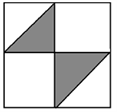if you observe carefully, you will realize that a square can be divided into 4 equal parts as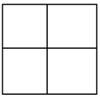And subsequently into 8 equal parts as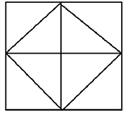The shaded regions can be represented in these equal regions as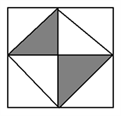The required shaded region forms 2 out of the 8 equal regions.

Hence, the area of the shaded region is (2/8)*82 = 16 sq cm

Based on this example, let us try and see how some common regular polygons can be Divided.

There are many ways to divide it, but we will look at the most common ways to divide it. Remember that this is for regular figures only, and also; there is more than one way to do any division.

1) TRIANGLES

A triangle can be divided into 2 equal parts in the following way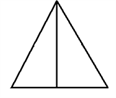A triangle can be divided into 4 equal parts in this way, where each part will have an area = (1/4)th of the total area.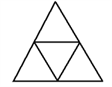It can be divided into 9 equal parts as follows, where each part will have an area = 1/9th of the total area.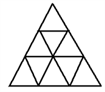And so on.

2) SQUARES

A square can be divided into 2 equal parts in the following ways.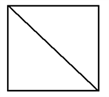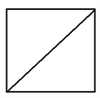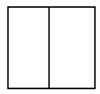It can be divided into 4 equal parts in one of the following ways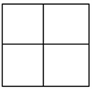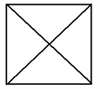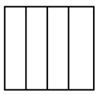Dividing a square into 8 equal parts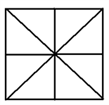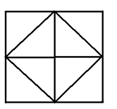It can be divided into 16 equal parts as follows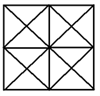Similarly, it can be divided into 3, 6,9 and 24 parts as follows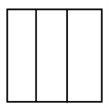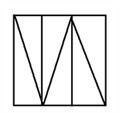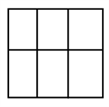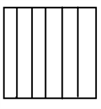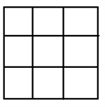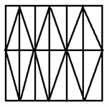3) HEXAGON

A hexagon can be divided into 6 equilateral triangles, with each triangle have an area =1/6th of the total area of the hexagon as follows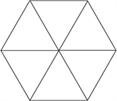A hexagon can also be divided into 3 equal rhombi as follows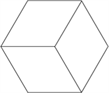It can also be divided into 12 equal parts in the following way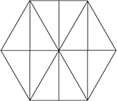Let us now look at the application of this technique with the help of some typical questions, which will help you understand Graphical Division completely.

Example 2

An isosceles right triangle is inscribed in a square. Its hypotenuse is a midsegment of the square. What is the ratio of the triangle’s area to the square’s area?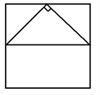Assume the square to have a side 2a. hence, area of square = 4a2

Using graphical division, we can divide the figure into 8 parts as follows. The triangle in question is the shaded part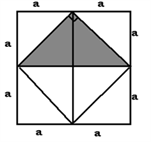Thus the triangle is 2/8th of the square area Hence ratio = 1:4

Example 3:

In the given diagram, ABCD is a rectangle with AE=EF=FB. What is the ratio of the area of the triangle CEF and that of the rectangle?

a) 1:4 b) 1:6 c) 2:5 d) 2:3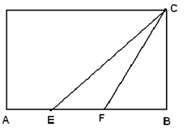The rectangle can be divided into 6 equal parts as follows, out of which CEF forms 1/6 of the total rectangle. Hence the ratio is 1:6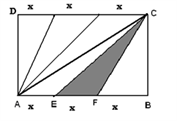Example 4:

In the figure given below, ABCD is a rectangle. The area of the isosceles right triangle ABE= 7 cm2, EC= 3BE. The area of ABCD (in cm2) is:

a) 21 b) 28 c) 42 d) 56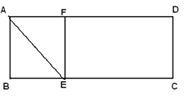Based on the data given in the question, if ABE is an isosceles right-angled triangle, then AB=BE=AF=FE. Then,

Area of ?AFE is also= 7 cm2 .also given that EC= 3BE.

Thus, we can graphically divide the figure as follows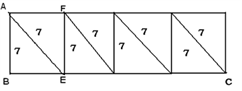The area of ABCD = 7*8 = 56cm sq.

Example 5:

ABCD is a square and E and F are the midpoints of AB and BC respectively. In the figure below find the ratio of ABCD:DEF?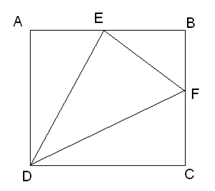SOLUTION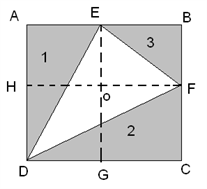FIGURE A

Let’s divide the figure using dotted lines as shown in Figure A.

Area of ABCD=100%.

Consider AEGD=50%. Then Area in the shaded region 1(AED)= 25%.

Similarly, DCFH=50%. The area in the shaded region 2 (DCF)=25%.

Now Area of EOFB= 25%. Area of shaded region 3(BEF)=12.5%. Total area outside triangle= 62.5%. Area inside triangle= 100-62.5=37.5%. required ratio = 100/(37.5) = 8:3

Example 6:

ABCDEF is a regular hexagon. Find the ratio of areas of ACE and ABCDEF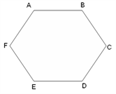SOLUTION:-

The required region ACE can be shown as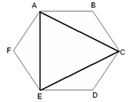A hexagon can be divided into 3 equal regions as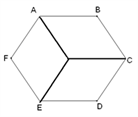The required region ACE can be easily drawn in this figure as follows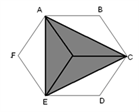The hexagon now comprises 6 equal triangles out of which 3 triangles form ACE. Thus, it can be clearly seen that ACE/ABCDEF= ½

Stay tuned with BYJU’S to get more notes for CAT exam preparation and get several sample papers, mock tests, and questions papers also. At BYJU’S, CAT aspirants are provided with complete information about the exam and are provided with complete assistance.